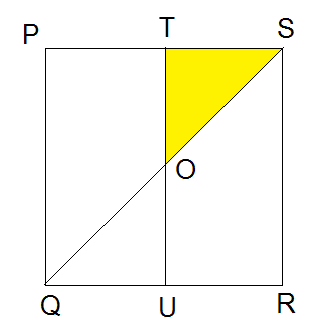# Just a Square

Geometry Level 1

$PQRS$ is a square. $T$ and $U$ are respectively the mid points of $PS$ and $QR$. Find area of $\Delta OTS$, if $PQ = 8 \text{ cm}$, where $O$ is the point of intersection of $TU$ and $QS$.×